101 Financial Ratios & Metrics To Improve Your Investing Skills - Sure Dividend

# Sure Dividend

## 101 Financial Ratios & Metrics To Improve Your Investing Skills

Updated on August 11th, 2021 by Bob Ciura

This article covers 101 financial ratios and metrics investors need to know.

This list is by no means all inclusive. It does however contain several interesting and informative metrics. You can use the links below to instantly jump to a specific section of the article.

Metrics are listed by category below:

The 8 Rules of Dividend Investing are a compilation of some of the most important metrics in this list. The 8 Rules of Dividend Investing help dividend growth investors build a portfolio of high quality dividend growth stocks trading at fair or better prices.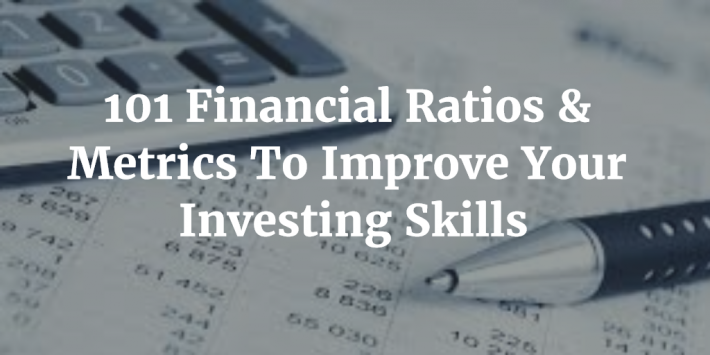### The Basics – Income Statement

The income statement has (arguably) the single most important financial report in today’s business world. It is a good starting point when analyzing a business.

### #1 Revenue

Revenue comes before earnings. Revenue is also known as sales or the ‘top line’. It is the amount of money generated from the sale of a product or service. If revenue is increasing that shows an increased demand for a company’s products/services. Declining revenue shows the opposite.

### #2 Expenses

Expenses are the opposite side of revenue. Revenue less expenses equals profit. Expenses are all the costs a business has (including taxes, interest, payroll, research and development, cost of goods sold, etc).

### #3 Depreciation

Depreciation is the reduction in value of an asset over time due to normal wear and tear. As an example, your car will be worth less next year than it is worth today. This decrease in value over time is expensed as depreciation in accounting. The depreciation of intangible assets is called amortization.

### #4 Earnings & Adjusted Earnings

You cannot calculate the price-to-earnings ratio without earnings. Earnings go by many names:

• Earnings
• Profits
• The-bottom-line
• Net profit

Earnings are what is left over after all expenses – including interest and taxes – are paid.

Earnings are a GAAP measure (generally accepted accounting principles). Corporations will often adjust earnings for 1 time or unusual expenses. These may include lawsuits, restructuring charges, or acquisition costs.

When used correctly adjusted earnings show a corporations ongoing earnings power. Corporate managements will sometimes use adjusted earnings to hide real problems in the business. Both earnings and adjusted earnings should be scrutinized before being blindly accepted.

### #5 Earnings-Per-Share

Earnings-per-share is total earnings dividend by total share count. It shows the amount of profit over the last 12 months that was generated for each share.

### #6 Gross Margin

Gross margin is gross profit divided by revenue. Gross profit is revenue minus cost of goods sold. Gross margin tells you what percentage of revenue a business keeps before paying any expenses other than the cost of goods.

### #7 Operating Margin

Operating margin is operating profit divided by revenue. Operating profit includes most expenses, but does not include interest or taxes. Operating margin gives picture of the profitability of a business without obscuring earnings power with interest expenses or differences in taxes.

### #8 Net Margin

Net margin (also called net profit margin) is net income divided by revenue. It is the ‘bottom line’ number that shows what percentage of every dollar in revenue a company keeps after accounting for all expenses.

### The Basics – Balance Sheet

The balance sheet shows the current position of a business, including cash, debt, and assets. It gives a snapshot of the financial state of a business.

### #9 Assets

An asset is property (including intangible property) that has value and could likely be used to meet debts, commitments, or liabilities. Examples include current assets, land, equipment, goodwill, patents, and vehicles (among many others).

### #10 Current Assets

Current assets are a subset of assets. The definition of a current asset is any balance sheet item that can be reasonably expected to be converted to cash within one year. Examples include: cash, cash equivalents, marketable investments (like publicly traded stocks), accounts receivable, and inventory, among others.

### #11 Liabilities

Liabilities are future obligations a business is likely to owe. They are the opposite of assets. Examples of liabilities include current liabilities and long-term debt.

### #12 Current Liabilities

Current liabilities are the opposite of current assets. Current liabilities are obligations that are reasonably expected to be paid within one year. Examples include short-term debt and accounts payable.

### #13 Debt

The term ‘debt’ is used interchangeably in accounting, finance, and investing. It often refers specifically to bonds, credit lines, and other borrowings. Occasionally debt is used as a synonym for liabilities, as is the case in the debt to equity ratio.

### #14 Equity

Equity is assets minus liabilities. It is a quick way to broadly gauge the overall built up value in a corporation. In general, more equity is better than less equity. Equity is also called book value.

### The Basics – Cash Flow Statement

The cash flow statement shows cash flows into and out of the company. It is less prone to management manipulation than the income statement.

### #15 Capital Expenditures

Capital expenditures are money spent by a business to purchase assets. Capital expenditures are often abbreviated as cap ex. It is important to differentiate between maintenance capital expenditures and growth capital expenditures. Maintenance capital expenditures should approximate depreciation (in straight forward cases) over the long run. Growth capital expenditures are funds spent on expanding the business rather than replacing old portions of the business.

### #16 Operating Cash flow & Cash Flow from Operations

Operating cash flow (also called cash flow from operations) is on the statement of cash flows, not the income statement. Operating cash flow shows a company’s cash flows from its normal operations. It does not include depreciation and amortization, as well as other non-cash charges.

### #17 Free Cash Flow

Free cash flow is calculated as operating cash flow minus capital expenditures. It is a cash based measure that does not suffer from the issues of accrual based accounting. Many investors prefer free cash flow to earnings as ‘cash doesn’t lie’.

The issue with free cash flow is determining the level of maintenance capital expenditures to growth capital expenditures. Free cash flow can unnecessarily penalize businesses which are investing heavily in growth. It is also more variable from year to year than earnings.

### #18 Market Capitalization

This is the total market value of a company’s outstanding shares.

### #19 Enterprise Value

Enterprise Value calculates what it would cost to completely take over a business.

To do so you would have to buy all the shares in a business (market cap) and extinguish all debt (total debt). Once you took over the business you could distribute all excess cash to yourself.

In its most basic form the formula for calculating enterprise value is: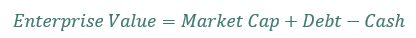Enterprise value has been shownto be superior to market capitalization when ranking stocks based on value.

### #20 Ticker

The ticker symbol (or ticker) is the 1 to 5 digit alphabetic code used to identify the shares of a specific corporation.

### #21 Sector

Sector refers to the broad business category a stock falls into. There is no standard sector classification for different sectors. The most common include the following:

• Basic Materials
• Consumer Goods
• Financial
• Health Care
• Industrial Goods
• Services
• Technologies
• Utilities

Sector lists sometimes break consumer goods into staples and discretionary categories. Energy is also sometimes separated from basic materials. Telecommunications is also sometimes given its own sector.

It is critical to have some diversification between sectors when building your dividend growth portfolio.

### #22 Volume

Volume is the amount of shares of a stock traded. It is generally calculated as daily volume. There is additional risk with buying and selling thinly traded shares as the bid-ask spreads tend to be much higher. Higher volume typically means you will be able to buy and sell easily.

### Profitability Ratios

Profitability ratios can be used to determine how efficient a business is at making money. They are also useful in comparing the profitability of different businesses to one another. In general (and there are plenty of exceptions – think Amazon as an example) higher profitability ratios mean a company has a stronger competitive advantage.

### #23 Return on Assets (abbreviated as ROA)

Return on Assets is calculated as net income divided by total assets. It is one of the simplest and most effective profitability ratios. Assets are used to scale profits as (virtually) every business has assets.

### #24 Return on Equity (abbreviated as ROE)

Return on equity is calculated as net income divided by equity. It shows the percentage of profit a company can make on its equity every year. For some businesses (a retailer in growth mode is a suitable example), this is a good way to gauge how quickly a company can grow.

### #25 Return on Invested Capital (abbreviated as ROIC)

Return on invested capital identifies the profit a company is making on money from its capital base.

The numerator in the formula is net operating profit after taxes (abbreviated as NOPAT). This does not include interest expense. The reason being that this ratio is looking to find profitability before payment to debt investors.

The denominator in the formula is invested capital. It is calculated as total assets less excess cash and non-interest bearing current liabilities. The reason excess cash is subtracted is because it is not being used in actively funding the business. Non-interest bearing current liabilities are subtracted because they are capital invested in the business by suppliers, not investors. This is basically ‘free capital’ and should not be included in the calculation. Accounts payable is a good example of non-interest bearing current liabilities.

### #26 Cash Return on Invested Capital (abbreviated as CROIC)

CROIC is very similar to return on invested capital. The denominator in this ratio is exactly the same as the denominator in ROIC.

The difference is in the numerator. ROIC uses NOPAT, while CROIC uses free cash flow in the numerator. Free cash flow is a cash based metric and is therefore not subject to the many estimates that go into accrual based measures like earnings.

### #27 Gross Profitability Ratio

Robert Norvy-Marx’s paper titled The Other Side of Value: The Gross Profitability Premium found that businesses with a higher gross profitability ratio outperform those with a lower gross profitability ratio.

Intuitively, this makes sense. A business that can earn high margins should have a strong competitive advantage in place to be able to resist market forces and charge such a high premium. Highly profitable businesses should make more money for shareholders than low profitability businesses, all other things being equal.

The gross profitability ratio is very easy to calculate. It is simply gross profits divided by assets. The higher the gross profitability ratio, the better. It is a quick way to compare the amount of gross profits a business can generate from its asset base.

### Stock Price Risk Metrics

Investing risk is fundamentally a qualitative exercise. To really understand the risk of a business you must understand its competitive position in the market and industry.

Qualitative investing is very messy. When opinions are involved there is no right or wrong before the fact. This makes creating a methodical framework for qualitative risk management difficult.

Perhaps because of this, several stock price based quantitative metrics have been designed to approximate investing risk.

### #28 Standard Deviation

Stock price (return series) standard deviation is the most commonly used risk metric. It is calculated as the annualized stock price standard deviation of a given security.

Stock price volatility is used as a measure for risk because businesses with uncertain futures should see their stock price fluctuate more wildly (as prospects change at the drop of a hat) versus stable businesses with more secure futures.

### #29 Beta

In most investing applications Beta refers to a specific securities’ sensitivity to overall stock market moves. A Beta greater than 1 shows more price sensitivity. That is to say, if the market declines by 10%, one would expect a stock with a Beta over 1 to decline by more than 10%. The converse is true on gains.

The higher the Beta, the riskier a stock is assumed to be. This is because it is more sensitive to the overall market than a stable business that is not so dependent on being in a positive market environment.

### #30 Maximum Drawdown

Maximum drawdown is the biggest decline a stock has suffered as measured from stock price high to stock price low.

Maximum drawdown is a useful measure because it shows the largest historical declines a stock has suffered. If a stock has historical maximum drawdowns of 50% and an investor cannot tolerate maximum drawdowns greater than 25%, they have no business investing in that security.

### #31 Value at Risk (abbreviated VaR)

Value at Risk is used to calculate minimum potential loss at a given confidence interval. VaR typically uses historical returns and the normal distribution. As an example, you could say at the 99% confidence interval (1% chance) my minimum expected loss is 20%. Said another way, I expect to lose 20% or more of the value of my investment 1% of the time.

### Fundamental Risk Formulas & Metrics

The ratios below take a different approach to quantitatively analyzing risk. Instead of looking at stock price movement, these formulas take data from a businesses’ financials to quantitatively measure risk.

### #32 Margin of Safety

The margin of safety concept is a risk management method popularized by Benjamin Graham. When one finds their estimated fair value of a company, they should not pay fair value. Instead, require a margin of safety so that if your fair value estimate is wrong, you still have margin of error in your purchase price.

Graham typically required a margin of safety of 67%. If his fair value calculation was \$10 per share, he would only pay \$6.70 for the stock so as to maintain his margin of safety.

### #33 Sloan Ratio

A 1996 study (which has been updated) by Richard Sloan at the University of Pennsylvania found that over a 40 year period (from 1962 through 2001) buying the lowest Sloan ratio stocks and shorting the highest Sloan ratio stocks resulted in compound returns of 18% a year.

The Sloan ratio formula is shown below: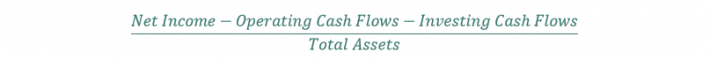The Sloan ratio is used to see if reported net income closely matches cash flows. If it doesn’t, net income may not accurately reflect business results.

• A Sloan ratio between -10% and 10% it is in the safe zone.
• A Sloan ratio from -25% to -10% or 10% to 25% is in the warnings zone.
• A Sloan ratio less than -25% or greater than 25% is the danger zone.

### #34 Piotroski F-Score

The Piotroski F-Score is a simple 9 point scoring system to separate successful businesses from unsuccessful businesses.

The 9 point scoring system is broken down into 3 categories:

Category 1: Profitability

1. One point if positive return on assets in current year
2. One point if positive operating cash flow in the current year
3. One point if higher return on assets in current year than previous year
4. One point if operating cash flows are greater than net income in current year

Category 2: Leverage, Liquidity, and Source of Funds

1. One point if long-term debt divided by assets is lower in current year than previous year
2. One point if current ratio is higher this year than previous year
3. One point if the company did not issue common shares in the current year

Category 3: Operating Efficiency

1. One point if gross margin is higher in current year than previous year
2. One point if asset turnover ratio is higher in current year than previous year

Investing in highly ranked F-Score stocks and shorting lowly ranked F-Score stocks resulted in 23% annual returns from 1976 to 1996.

The F-Score works by identifying businesses with cash generating operations – that are also seeing operations improve.

### #35 Altman-Z Score

The Altman-Z Score was first introduced in 1968 by Edward Altman to estimate bankruptcy risk for manufacturing firms.

In 2012 he reintroduced the formula and provided an update (called the Altman-Z Plus Score) for any type of firm (not just manufacturing concerns). The formula for the Altman-Z Plus Score is below: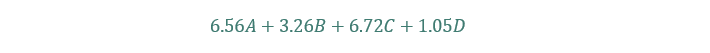• A = Working Capital divided by Total Assets
• B = Retained Earnings divided by Total Assets
• C = EBIT divided by Total Assets
• D = Book value dividend by Total Liabilities

If the Altman-Z Score is above 2.6, the firm is likely financially sound.

If the Altman-Z Score is below 1.1, the firm is likely to go bankrupt.

The average Altman-Z Score of non-bankrupt companies is 7.7.

### #36 Beneish-M Score

The Beneish-M Score is used to determine if a company is manipulating its earnings. Catching earnings manipulators early can save investors tremendous sums of money (the most famous earnings manipulator is Enron).

The original M-Score includes 8 variables. An updated version includes just 5 variables but performs slightly better than the 8 variable version.

• Days sales in receivables Index (abbreviated as DSRI)
• Gross margin index (abbreviated as GMI)
• Asset quality index (abbreviated as AQI)
• Sales growth index (abbreviated as SGI)
• Depreciation index (abbreviated as DEPI)

Each index metric is calculated as (Metric in current year) divided by (metric in prior year).

Each metric is given a corresponding weighting to calculate the Beneish-M Score. The formula is below: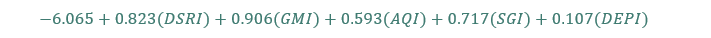If a company’s score is greater than -1.78 then there is a high likelihood the company is manipulating its earnings. The more negative the score, the better.

### Risk/Return Ratios

Analyzing performance based upon returns alone does not factor in the amount of risk taken to acquire those returns. The risk/return ratios below all take different approaches to better quantifying investment performance while taking into account both risk and reward.

### #37 Sharpe Ratio

The most widely used risk/return ratio is the Sharpe ratio. The Sharpe ratio is shown in the image below: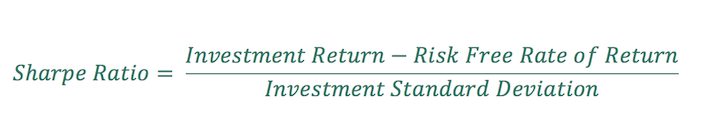The Sharpe ratio subtracts the risk free rate of return from the return of the asset in question. This shows the excess return; the return above what you could have made from investing in a ‘riskless’ asset. The riskless asset is usually approximated via T-bill (short term United States government debt obligations with maturities less than 1 year) returns.

Excess return is then divided by the standard deviation of the return series. This divides returns by a proxy for risk. The more volatile returns are, the riskier they are said to be.

### #38 Treynor Ratio

The Treynor ratio is identical to the Sharpe ratio except it uses Beta instead of standard deviation as the measure of risk.

The Treynor ratio is appropriate to use when a portfolio has diversified away non-systematic risk and has only systematic risk remaining. An example would be a well-diversified stock mutual fund.

The formula for the Treynor ratio is shown below: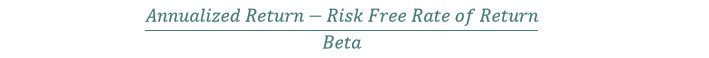### #39 Sortino Ratio

The Sortino ratio seeks to improve on the Sharpe ratio by better defining risk. The Sortino ratio only looks at the downside standard deviation of returns. This means that upside volatility (positive returns) do not impact risk.

This is logical in that most investors are very happy to see their stocks jump 20% in one day, even though this would increase the standard deviation of the return series and increase risk according to the Sharpe ratio. The Sortino ratio does not suffer from this flaw.

The formula for the Sortino ratio is below:### #40 Calmar Ratio & MAR Ratio

The Calmar & MAR Ratios are very similar. They both uses Maximum Drawdown as the risk measure instead of standard deviation. Both also do not take the risk free rate of return into account.

Where the Calmar & MAR ratios differ is the time period in which they calculate returns and maximum drawdowns.

The Calmar ratio uses 3 years of rolling data. The MAR ratio uses data since inception of the investment/portfolio/account.

The formula for the Calmar Ratio is below:The formula for the Mar ratio is below:### #41 Sterling Ratio

The Sterling ratio is very similar to both the Calmar and MAR ratios. The Sterling ratio takes into account the idea that the largest historical drawdown is not the largest possible maximum drawdown.

The Sterling ratio has an arbitrary ‘+10%’ added to the largest maximum drawdown to account for potentially larger future drawdowns.

The formula for the Sterling ratio is below:### #42 Omega Ratio

To calculate the Omega ratio, one must first pick a target return threshold. A common target is either 0% or the risk free rate (when calculating the Omega ratio).

The Omega ratio is calculated by summing historical returns above the return threshold minus threshold return and dividing them by the absolute value of the sum of returns below the threshold minus threshold return.The Omega ratio can be used on non-normal distributions. This gives it a distinct advantage over ratios that use standard deviation. Stock price returns approximate the normal distribution, but they are not actually normally distributed. There are far too many ‘outlier events’ (think Black Monday in 1987) than a normal distribution would predict.

### #43 Information Ratio

The information ratio measures a portfolio’s consistency and returns relative to a benchmark.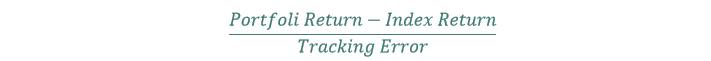A high information ratio is achieved by building a portfolio that:

1. Closely tracks an index
2. Significantly outperforms an index

This is very difficult. A high information ratio shows that a portfolio manager is sticking with the outlined strategy while adding significant value when making investment decisions that differ from the index.

### #44 Upside & Downside Capture Ratios

The downside capture ratio measures how a portfolio performed versus a benchmark when the benchmark fell in value.

The upside capture ratio measures how a portfolio performed versus a benchmark when the benchmark rose in value.

Capture ratios are calculated by dividing the portfolio performance over a time period by the benchmark performance over the same time period.

In an ideal world your portfolio would capture all of the upside movements of the market and none of the downside movements.

### Dividend Ratios & Metrics

There are several metrics suited specifically for dividend investors. There are many reasons to be a dividend investor. Chief among them is that dividend growth investing has historically outperformed the market – with lower stock price standard deviation. Secondly, dividend growth investing provides rising dividend income over time, which is important for investors seeking steady income in retirement (or early retirement).

### #45 Dividend Yield

Dividend yield is a company’s dividend payments per share divided by its share price. It is one of the most used metrics in dividend investing. All other things being equal, the higher the better. You can see an extensive list of high dividend stocks here.

### #46 Dividend Payout Ratio

The Dividend payout ratio is a company’s dividends divided by earnings. The higher the payout ratio is the larger the percentage of earnings being used to fund the dividend. By definition a payout ratio above 100% is unsustainable.

### #47 Dividend Payback Period

The dividend payback period calculates the number of years it will take a dividend growth stock to ‘pay back’ the initial purchase price. The dividend payback period can be calculated with:

• Stock price
• Expected growth rate
• Annual dividend payment

The lower the dividend payback period, the better. The dividend payback period is not easily calculated like some of the other metrics in this article (like the P/E ratio, for example). You can download an Excel spreadsheet that quickly calculates the dividend payback period for a dividend growth stock at this link.

### #48 Yield on Cost

Yield on cost measures the percent of dividend income your investment is generating from the purchase price.

If you buy a stock with a 3% dividend yield, and then annual dividend payments double over the next 10 years, your yield on cost will be 6%.

Warren Buffett’s investment in Coca-Cola has a yield on cost of around 50%. He is getting back about 50% of his original investment in Coca-Cola every year from dividends.

Businesses with strong competitive advantages combined with years of growth create large yields on cost.

### #49 Dividend Discount Model

The formula for the dividend discount model is shown below:The dividend discount model is used to quickly estimate the ‘fair value’ of a dividend growth stock. An example is below.

Imagine a company is expecting \$1.00 in dividends-per-share next year. The appropriate discount rate is 10%, and the growth rate is 5%. This stock’s fair value according to the dividend discount model would be \$20.

The beauty of the dividend discount model is its simplicity. The difficulty in applying it practically is coming up with a ‘fair’ discount rate and an accurate future growth rate.

With that said, the dividend discount model is a useful tool for coming up with a ‘ballpark estimate’ of fair value for dividend growth stocks that have strong competitive advantages.

### #50 Dividend History

Dividend history is simply the amount of time a business has paid dividends. This may seem like a vanity metric, but dividend history matters.

Businesses with 25+ years of rising dividends are less likely to cut their dividend payments.

There are several interesting groups of stocks by dividend history.

### Growth, Return, & Performance Ratios & Metrics

Not enough investors know about the different ways of calculating growth. There is a big difference between the arithmetic growth rate and the geometric growth rate

### #51 Arithmetic Growth Rate

The arithmetic growth rate is the simple average of returns. As an example, let’s assume a stock has the following returns over 3 years:

• Up 25%
• Down 50%
• Up 25%

Under these assumptions the arithmetic average growth rate is 0% per year. You might think you came out at break-even using the arithmetic growth rate, but that is not the case…

In reality your portfolio would be down 22%.

This is explained in the geometric growth rate section below.

### #52 Geometric Growth Rate

The geometric growth rate is also called the compound growth rate or the time series growth rate.

Remember our example from above? In the real world, compounding matters. The geometric growth rate takes into account compounding, also called growth through time.

Imagine you have the following annual returns:

• Up 25%
• Down 50%
• Up 25%

To calculate the geometric growth rate you would do the following: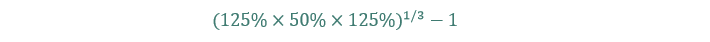This comes to -7.9% a year, which is a decidedly poor return (and not even close to the 0% growth rate the arithmetic average would’ve calculated).

The formula for using the geometric growth rate is below:### #53 Total Return

Total return is stock price appreciation (or depreciation) plus dividend payments. It is the total return from an investment, including capital gains and dividends.

Total return is typically used when calculating performance as dividends are often a large portion of total returns.

### #54 Survivorship bias

Survivorship bias is a common error in thinking and studies. It is when you look only at the surviving stocks in a study, and not at the ones that dropped out.

As an example, if you were to calculate the return of all Dividend Aristocrats since 2000 and only looked at the ones that are still Dividend Aristocrats you would have survivorship bias in your study. This is because the study did not take into account the stocks that were Dividend Aristocrats, but cut their dividends between year 2000 and now.

### Valuation Ratios, Metrics, and Formulas

There is no one ‘correct’ way to value a business. There are however, a wide variety of financial ratios and metrics that can be used to compare the relative value of stocks to one another.

### #55 Price-To Earnings Ratio

The price-to-earnings ratio is one of the most important investing metrics to know. It is a quick way to broadly gauge the sentiment around a stock.

The higher the price-to-earnings ratio, the more you must pay for \$1 of a company’s earnings. All other things being equal, a high price-to-earnings ratio signals the market expects rapid growth from a company while a low price-to-earnings ratio signals expected low or negative growth.

At a price-to-earnings ratio of 20, you must pay \$20 for every \$1 of annual earnings from the company. The price-to-earnings ratio is calculated as share price divided by earnings.

### #56 Enterprise Value to EBITDA

There is significant evidence that the Enterprise Value to EBITDA ratio is one of the two best valuation metrics. It has historically outperformed the following metrics:

• Price-to-earnings
• Enterprise value to free cash flow
• Enterprise value to gross profit
• Price-to-book

It appears that using enterprise value instead of market cap (which is the same as price) in the denominator of value ratios improves results. This is likely because enterprise value takes into account total capital structure, including debt and cash. In its simplest form, enterprise value is calculated as follows:Businesses with large amounts of cash on their balance sheet and no debt score better with enterprise value than they do with market capitalization.

Using EBITDA is also beneficial. EBITDA is perhaps the best measure of showing how much cash a business is generating irrespective of capital structure, taxation, and depreciation.

The biggest downside to EBITDA is that it does not account for depreciation. This is rather troubling, as depreciation is a real part of cash flows. Using EBIT in place of EBITDA is preferable from a conceptual standpoint.

### #57 Enterprise Value to EBIT

Not only is using EBIT in place of EBITDA preferable from a conceptual standpoint, it is also preferable from a historical return standpoint.

In the book Quantitative Value, Tobias Carlisle and Wesley Gray demonstrate that the Enterprise Value to EBIT multiple has outperformed the EBIDTA to Enterprise Value multiple (and all other valuation metrics) from the period 1964 to 2011.

For investors looking for a single valuation metric, Enterprise Value to EBIT is likely the best.

### #58 Enterprise Value to Free Cash Flow

This metric is similar to the two above. Instead of using EBIT or EBITDA in the denominator, it uses free cash flow. Free cash flow comes from the statement of cash flows rather than from the income statement and is preferable when one believes a company may not be correctly stating earnings. Cash doesn’t lie.

### #59 Price to Sales

The price to sales metric uses the very first item on the income statement – sales. The advantage the price to sales ratio has over others is that it works for virtually all businesses. Not all businesses are profitable, but nearly all have sales.

The price to sales ratio helps to compare businesses that may not be profitable currently, or that are experiencing a temporary decline in profit margins.

### #60 Price to Book Value

The price to book ratio compares the price of a stock to its book value. This ratio works well for businesses that rely on assets or equity to produce cash flows. It is not well suited for franchises, and does not work at all on businesses with negative equity.

### #61 Price to Tangible Book Value

Tangible book value is book value minus intangible assets and goodwill. It looks only at ‘real’ assets and ignores goodwill and other intangibles. The price to tangible book value ratio can be used in place of the price to book value ratio when one feels that a company’s intangible assets are obscuring the true value of assets.

### #62 Forward Price to Earnings Ratio

The forward price to earnings ratio divides the current price by next year’s expected earnings. The ratio is useful when a business’s current year earnings are significantly understated or overstated by large one time events.

### #63 Price to Earnings to Growth Ratio (abbreviated PEG)

The PEG ratio was popularized by Peter Lynch. It is calculated as follows: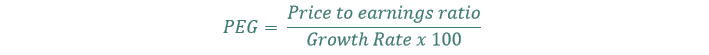The PEG ratio takes into account growth rate when considering valuation. This is intuitive. A company growing at 10% a year should have a higher price-to-earnings ratio than a company growing at 2% a year. The PEG ratio takes this into account.

A PEG ratio below one is generally said to be a bargain.

### #64 Modified Price to Earnings to Growth Ratio

The PEG ratio does not take into account dividend payments. Dividends can be a large part of total returns. The modified PEG ratio does take dividends into consideration.

The formula for the modified PEG is below:### #65 Shiller Price to Earnings Ratio

The Shiller price to earnings ratio (also called PE10) uses average earnings over the last 10 years instead of trailing twelve months earnings in the denominator.

This technique is beneficial for cyclical businesses or businesses with rapidly fluctuating earnings. This site has a very long-term perspective on the S&P 500’s PE10 ratio.

### #66 Negative Enterprise Value

Unlike the preceding valuation tools, negative enterprise value is not a ratio. A business has a negative enterprise value when it has enough cash on its books to completely pay off all its debt AND buy back all its shares.

This does not (or very, very, rarely) happens to profitable businesses. If you identify a business with a negative enterprise value, it is ripe for a takeover or acquisition. If the business were to be acquired, all the cash could be distributed to shareholders and the business be shut down, which would result in positive returns with very little risk.

You can see a list of negative enterprise value stocks at this link. Be sure to check data yourself, and use a screener as the ‘first step’ in the investing process. Always do your due diligence.

### #67 Discount to Net Current Asset Value

Net current asset value is calculated as current assets minus total liabilities.

Net current asset value is commonly abbreviated as NCAV.

Benjamin Graham generated returns of around 20% a year over several decades by investing in a diversified portfolio of companies trading at 67% or less of their NCAV.

The idea behind NCAV is if a business is trading for less than the value of its current assets less all liabilities, it is very certainly undervalued. Benjamin Graham wanted a large margin of safety (hence the 67% of NCAV) on this type of investment.

In today’s market, there are very few NCAV stocks available. NCAV stocks become more common during deep bear markets.

### #68 Discounted Cash Flow

Discounted cash flow analysis is a method of finding the ‘fair value’ of a business. Discounted cash flow analysis is the correct way to value an investment if you have 100% perfect information on the future.

Discounted cash flow analysis discounts the sum of all future cash flows of a business back to present value using an appropriate discount rate.

Assuming you have misplaced your crystal ball and your psychic powers have stopped working, discounted cash flow analysis has serious flaws because it requires so many assumptions.

Discounted cash flow analysis requires the following assumptions:

• Discount rate
• Growth rate
• When earnings stream will start and stop

Because of this the fair value derived from discounted cash flow analysis. With that said, the metric does have utility in bringing to forth the assumptions you are making in your valuation, and how it effects the total value of a stocks.

### #69 Earnings Yield

The earnings yield is the inverse of the price-to-earnings ratio. It shows what percentage of money would be returned to you by a company at a current price if you owned the business and distributed 100% of net profit.

### #70 Magic Formula

The magic formula was popularized by successful Hedge Fund manager Joel Greenblatt in The Little Book That Beats the Market.

The Magic Formula ranks stocks on 2 metrics:

• Rank based on EBIT/Enterprise Value
• Rank based on EBIT/(net fixed assets + working capital)

The first ranking signal in the magic formula works very well. The second signal ads no value and actually decreases returns from the first signal as evidenced in the book Quantitative Value.

The idea behind the Magic Formula is to find:

The EBIT/Enterprise Value metric works well to find undervalued businesses.

The other metric (which Greenblatt refers to as Return on Capital) does not work well in identifying businesses with strong and durable competitive advantages.

It is also important to note that Greenblatt’s claims of 30% annual returns from the magic formula re disputed and cannot be independently verified by other historical studies.

### #71 Net Net Working Capital

Net Net Working Capital stocks are business trading below liquidation value. They are extremely cheap (and often for good reason).

When a business trades for below liquidation value, a little good news can send the stock surging upwards as it is priced for nothing but negativity.

The formula to calculate net net working capital (abbreviated as NNWC) is below:Benjamin Graham created and popularized NNWC investing. NNWC capital stocks are very rare in today’s investing world. NNWC stocks tend to provide very high returns over time (similar to NCAV and Negative Enterprise Value stocks).

NNWC investing is a form of deep value investing.

### #72 Shareholder Yield

Shareholder yield shows how much cash an investment is returning to shareholders as compared to its price.

Shareholder yield (in its most basic form) is calculated as:Businesses with high shareholder yields show that the company is:

1. Trading at a low price relative to cash returned to shareholders
2. Has a shareholder friendly management that looks to reward shareholders with cash

### #73 Graham Number

Benjamin Graham pioneered value investing. As a result, many of the metrics in the valuation section come from him.

The Graham number looks to find the maximum acceptable price for a well-established business. The Graham number is calculated as:The Graham number finds the maximum fair value to pay for a business. As an example, if a company has \$1 in earnings-per-share and \$5 in book-value-per-share, the Graham number would be \$10.61. If the stock was trading for under \$10.61 a share it would be a buy (though Graham would likely look for a margin of safety on top of this).

### Balance Sheet & Debt Risk Metrics

The ultimate risk facing any business is insolvency. Having a high debt burden makes a business going bankrupt more likely because it must constantly pay creditors. The metrics below take a variety of approaches to looking at a businesses’ ability to handle its debt burden.

### #74 Equity to Assets Ratio

The equity to assets ratio shows the percentage of assets owned by the company.

The formula to calculate the equity to assets ratio is equity divided by assets.

The higher the equity to assets ratio, the greater percentage of the company’s assets that are owned by the company and not from debt purchases.

### #75 Cash Flow to Debt Coverage Ratio

This ratio is calculated as operating cash flows divided by total debt.

The higher the ratio, the quicker a company can pay off its debts. If the ratio is above 1, the company could use its cash flows to pay off its debt in under a year.

### #76 Quick Ratio

The quick ratio is used to determine a company’s short term liquidity situation.

It is calculated as:The quick ratio is designed to show if a company is able to meet its short term liabilities. If the quick ratio is below 1, the company is at serious risk of bankruptcy as it does not have enough cash on hand to pay debts coming due in the next year.

The higher the quick ratio, the better.

The quick ratio is also known as the acid test ratio.

### #77 Debt to Equity Ratio

The debt to equity ratio is calculated as total liabilities divided by equity.

The ratio is used to calculate how leveraged a company is relative to its owned value (as measured by equity).

Highly leveraged businesses are at greater risk of insolvency as they must continuously meet their debt holder payment obligations.

### #78 Interest Coverage Ratio

The interest coverage ratio is commonly calculated as EBIT divided by interest expense.

The higher the interest coverage ratio, the better.

The interest coverage ratio shows how well covered a company’s interest expenses are by its earnings before interest and taxes.

Any business with an interest coverage ratio below 1 is in serious danger.

An interest coverage ratio above 1.5 is the threshold for ‘not at immediate risk’. Most stable businesses have interest coverage ratios far higher than 1.5.

### Technical & Momentum Ratios and Indicators

The metrics below use stock price data to determine optimal entry and exit points for investing.

### #79 52-Week Range

52 week range is the high and low price of a stock over the last year. As an example, if a stock had a high price of \$60 over the last year and a low price of \$40 over the same time period, it’s 52 week range would be \$40 to \$60 per share.

Momentum investors typically look to buy near the 52 week high, while value investors will be more interested in stocks trading near the 52 week low.

### #80 Momentum

Momentum can be measured in a large variety of ways. At its core it is a measure of the past performance of a stock.

Positive momentum has been shown to produce market beating returns over the next month. The first widely credited large study on the subject was done by Jegadeesh and Titman in 1993.

The most widely used measure of momentum is 12 month performance, skipping the most recent month (11 months of performance data). This is because the first month is associated with mean reversion and is therefore not included in momentum calculations.

While momentum has been shown to produce excess returns on par with value investing (and even outpacing it), momentum investing is not suitable for long-term investors as it involves significant buying and selling (in general; there are momentum based asset class strategies with lower turnover).

### #81 Simple Moving Average

The simple moving average is a metric often used to determine when an asset should be held, and when it should be sold.

The most common simple moving average used is the 200 day simple moving average. Securities that are trading above their 200 day simple moving average have been shown to have higher returns than when trading below their 200 day simple moving average. This is very likely the same (or similar) effect that is picked up with past performance momentum.

### #82 Relative Strength Index (abbreviated RSI)

The relative strength index compares recent gains to recent losses. The goal of the relative strength index is to determine if a security is overbought or oversold.

The formula for the relative strength index is below:RSI ranges from 0 to 100. When the RSI is 70 or above the security is said to be overbought. When the RSI is 30 or below it is said to be oversold.

### #83 Average True Range

Average true range is often abbreviated as ATR.

The average true range is another way to measure the volatility of a security.

Average true range is calculated as a simple moving average (often 14 days) of a company’s true range.

True range is calculated as the highest of the following:

• High in a period minus low in a period
• Absolute value of period high minus previous close
• Absolute value of the period low minus previous close

### Capital Asset Pricing Model & Portfolio Ratios & Metrics

The metrics below are used in modern portfolio theory and the capital asset pricing model. In addition, metrics used to examine portfolio characteristics are included in this section.

### #84 Alpha

Alpha is ‘excess return’. It is return greater than what one would expect from an investment using the capital asset pricing model. High positive alpha shows one is outperforming the market while controlling for risk (as measured by Beta).

### #85 Risk Free Rate of Return

The risk-free rate of return is the return you could generate from a ‘riskless asset’.

The term is a bit of a misnomer as no asset is truly risk free.

The proxy most often used for the risk free rate is the yield on Treasury Bills. The 1 year treasury bill currently has a yield of 0.08%.

### #86 WACC

WACC stand for weighted average cost of capital.

The WACC shows the blended cost of debt and equity financing for a company. The formula for WACC is below:The cost of equity is the average investor expected return from the common stock. This is unknowable. In practice. The most common way to calculate the cost of equity is to use the capital asset pricing model. The formula for the capital asset pricing model (abbreviated as CAPM) is below: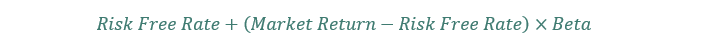Example:

• Risk free rate is 4%
• Expected market return is 9%
• Beta is 1.5

In this case, the stock’s cost of equity is 11.5%.

Calculating the cost of debt is easier. The cost of debt is the weighted average interest rate on debt. Since interest rates reduce taxes, a tax shield adjustment is made on the cost of debt. This is the (1-Tax Rate) portion of the WACC formula.

Continuing with our example:

• Cost of equity is 11.5%
• Equity financing is 60%
• Debt financing is 40%
• Cost of debt is 8%
• Tax rate is 30%

In this case the WACC is 9.14%.

The WACC is useful in determining what projects a company should take on. A business should never take on projects with a projected rate of return below the WACC.

### #87 R-Squared

R-squared is a measure of how well data fits a linear regression. R squared ranges from 0% to 100%. 100% is a perfect fit, while 0% means your model does not explain your data at all.

In investing R-squared measures how much of a fund or portfolios returns can be explained by underlying market movement.

### #88 Active Share

Active share measures how different a fund or portfolio’s holdings are from the benchmark. The greater the difference, the higher the active share.

Higher active share is somewhat correlated with outperforming the market. One cannot hope to outperform a benchmark by much if active share is low, as the portfolio is too similar to the benchmark.

### #89 Tracking Error

Tracking error shows the difference in performance between a fund or index and its benchmark. For ETFs, a large tracking error is negative because the ETF is not tracking the benchmark it should be.

### #90 Correlation

Correlation measures how securities move together.

Correlation ranges from -1 to 1. A score of -1 is a perfect inverse relationship. A score of 1 is perfect relationship; the securities move in lock-step with each other. A score of 0 shows no relationship at all.

Investing in a wide variety of securities with positive expected returns and low correlations is the goal of a diversified portfolio. In practice, this is very difficult as most asset classes see their correlations converge when you need them not to – during market corrections.

### Alternative Earnings Metrics

Earnings is not the only want to calculate money ‘to the good’ a business generates. This section looks at several alternatives to earnings that investors can use to track how much money a company is making.

### #91 Owner’s Earnings

Owner’s earnings is the earnings metric Warren Buffett uses. To calculate owner’s earnings, do the following:

2. Add back depreciation and amortization
4. Subtract maintenance capital expenditures
5. If working capital increased, subtract change in working capital
6. If working capital decreased, add change in working capital

The difficult part of calculating owner’s earnings is finding maintenance capital expenditures.

Maintenance capital expenditures are not a normal part of financial statements. Investors must dissect capital expenditures and estimate how much was used for growth and how much for maintenance.

### #92 FFO

FFO stands for funds from operations.

This metric is commonly used for REITs instead of earnings. Since REIT’s assets are their primary business, depreciation significantly impacts results. Depreciation accounting rules often do not match up with real world depreciation. This makes FFO necessary.

FFO is calculated as net income excluding gains or losses on the sale of property, with depreciation added back in. FFO is a much better profitability measure for REITs than earnings.

### #93 AFFO

AFFO stands for Adjusted Funds From Operations.

It takes funds from operations and adjusts for recurring capital expenditures, as well as other adjustments from management. AFFO is typically the most representative measure of ‘real earnings’ from REITs.

### #94 Short Ratio

The short ratio is also called short float. It shows the percentage of tradeable shares being sold short. The higher the short ratio, the more investors are betting a stock’s price will fall. Stocks with high short ratios tend to have serious questions regarding their underlying business model.

### #95 Insider Ownership

Insider ownership is the percentage ownership in a business by the following:

• Shareholders with more than 10% ownership of the company
• Officers and directors of the company

### #96 Institutional Ownership

Institutional ownership is the percentage of ownership of a stock by large ‘sophisticated’ investors such as hedge funds, ETFs, mutual funds, private equity funds, and pension funds.

Large institutional ownership generally means a stock will be well covered. On the downside, high institutional ownership can lead to big swings in a stock’s price as institutional investors tend to buy and sell together.

### #97 Cash Conversion Cycle

The cash conversion cycle is used to measure how quickly a company can convert cash on hand into even more cash on hand.

The cash conversion cycle is broken down into 3 parts:

• Days Sales of Inventory (abbreviated as DSI)
• Days Sales Outstanding (abbreviated as DSO)
• Days Payable Outstanding (abbreviated as DPO)

The cash conversion cycle is calculated as DSI + DSO – DPO.

### #98 Days Sales of Inventory

Days sales of inventory measures the average length of time a company’s cash is tied up in inventory before it is sold.

Days sales of inventory is calculated as: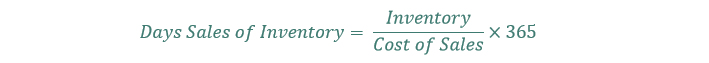The lower the days sales of inventory is, the quicker a business can convert its inventory into sales.

### #99 Days Sales Outstanding

Days sales outstanding calculates how long a company takes to collect on its sales. Days sales outstanding is calculated as: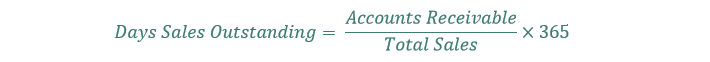The lower the days sales outstanding is, the faster a company can collect on its payments.

### #100 Days Payable Outstanding

Days payable outstanding measures how long a company can wait before paying back its creditors. It is calculated as follows:The higher the days payable outstanding, the more free credit a company can squeeze out of its supplies.

### #101 Inventory Turnover Ratio

Inventory turnover ratio shows how many times in one year a company’s inventory is being replaced. The higher the inventory turnover ratio, the quicker inventory is ‘flying off the shelves’, and (in general) the more demanded a company’s products are.

The inventory turnover ratio is calculated as sales divided by average inventory in a period.